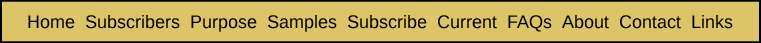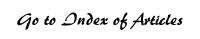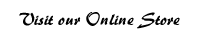Derivation of Formulas for the Probability of a Given ELS, Which Only Occurs Once, of Crossing a Specific Section of Text Appendix Four from Breakthrough: Encountering the Reality of the Bible Codes In this appendix, our goal is to turn the discussion in the previous appendix into the derivation of algebraic formulae that can be applied in any given example. In the last appendix, we dealt only with one specific example. In it, the length of the total text (T) was 50, the length of the ELS (L) was three, the number of skips, the width (W) of the section of text was three (letters) and that section consisted of the 30th, 31st and 32nd letters in Lincoln's Gettysburg Address. Our goal is to derive a formula for the probability that a given ELS, which only occurs once in the entire text, will cross a specific section of text. As in the example from the last appendix, this will be derived as the ratio of the total number of ELSs that could cross the specific section of text to the total possible number of ELSs that can occur within the entire text. We already have a formula for the denominator when the interval can be any number between 1 and N. It is given as Formula 1C: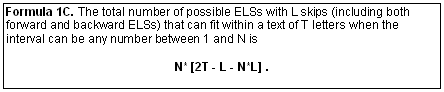So our quest will be reduced down to deriving a formula for the numerator. While this will not involve anything more than high school algebra, it will get a bit protracted and messy. In large part this is due to the fact that the formula for the number of ELSs with a given interval (i) that can cross a specific section of text varies substantially from one "region" to the next. And there are three regions, or ranges of the possible intervals for the ELS. As a practical matter, however, once these formulas are put into a spreadsheet, calculations become quick and easy. We will begin by introducing some more algebraic symbols. The number of the first letter of the section of text (30 in our example) will be denoted by the letter b, for beginning. And the number of the last letter of the section of text (32 in our example) will be denoted by the letter e, for ending. Let us first consider the number of letters encompassed by the beginning of the text (letter 1) and the first letter of the given section of text (letter b). This number is b itself, because we started counting the letters with the first one and continued counting until we got to the number of the first letter of the section of text. Next consider the number of letters encompassed by the last letter of the section of text (e, or 32) and the last letter of the entire text (T, or 50). That number is T – e + 1. This is because we actually started counting at (e – 1), or 31, as being 0, so that 32 is equivalent to 1, or the first letter in our sequence. This was described earlier in Appendix Three. So we have two sequences of letters, the first consisting of b letters, beginning with the first letter of the entire text and ending with the first letter (b) of the given section of text. And we have a second sequence of letters commencing with the last letter of the given section of text (e) and ending with the last letter of the entire text (T). We will now define two new variables for the sake of simplifying subsequent formulas we will derive. The first will be the number of letters (c) in the shortest of the above two sequences. It will be defined by the formula c = minimum (b, T – e + 1). The second will be the number of letters (d) in the longest of the above two sequences. It will be defined by the formula d = maximum (b, T – e + 1). In our example, c = minimum (30,19) = 19, and d = maximum (30,19) = 30. Recall that the length L of an ELS is defined as the number of skips it contains. L will be equal to the number of letters in the ELS minus one. If an ELS has four letters, then it has three skips, because the first skip is the one between the first and the second letter. The second skip is the one between the second and the third letter and the third one is the one between the third and the fourth letter. If the interval of the ELS is 1, then no letters are included in any of the skips. The interval is the amount by which the number position of successive letters of the ELS increases. If the interval is 2, then one letter occupies each skip position. For example, if an ELS of four letters starts in position 20 and has an interval of 2, then it occupies positions 20, 22, 24 and 26. This ELS has three skips, and they consist of the positions 21, 23 and 25. We are now in a position to quickly define the boundaries of each of the regions R0, R1 and R2 introduced in the last appendix. These regions consist of ranges of values of the intervals where certain conditions exist. You may recall from Appendix Three that the first region R0 consists of interval values where no ELS can possibly reach beyond either of the boundaries of the complete text. In other words, no ELS can reach all the way from the given section of text to a letter position beyond either the beginning or the ending of the complete text. We will see that R0 is defined by i < c / L. In the example from the last appendix, c = 19 and L = 3, so that c / L is 6 1/3. So we have i < 6 1/3. This gives us i values of 1 through 6, as is shown in Table 3A. Notice in that table that if T had been only 49, instead of 50, then c = 18 and i would have to be less than 6, so that R0 would only consist of interval values of 1 through 5. If we look at Table 3A, for interval 6, we see that the last crossing occurs with the ELS occupying positions 32, 38, 44 and 50. If T had only been 49, then this ELS would extend beyond the last letter of the entire text. In this case, the interval of 6 would belong to Region R1, where at least one ELS can reach all the way from the given section of text to a letter position beyond either the beginning or the ending of the complete text. This suggests that we can define R1 by the following relationships: (c / L) < i < (d / L) In the Appendix Three example, d / L is 30 / 3 = 10. This gives us 6 1/3 < i < 10. In our example, R1 would consist of intervals greater than or equal to 6 1/3 and less than 10. This would be satisfied by interval values of 7, 8 and 9. This can also be seen by reviewing Table 3A. Finally, R2 is defined by (d / L) < i < M. So R2 is defined by interval values that are greater than or equal to 10. In Table 3A, R2 consists of interval values of 10 through 16, with 16 being defined by the maximum possible interval. In order to have the definition of R2 change in our example, d would have to get down to 27 (rather than being 30) in order for the first interval in R2 to be 9. In that case, d / L would be 9 and so the smallest interval in the region could be 9. In deriving various formulas in the rest of this appendix, it will be helpful to define the following values of i: M0 = the largest value of i in region R0. M1 = the largest value of i in region R1. M2 = the largest value of i in region R2. We can summarize all of the above discussion in the first two columns of the following table: Table 4AThe formula for the number of crossing ELSs for R0 is a composite of W+(L*i) for values of i from 1 to W and of W+(L*W) for values of i from W to M0. While there are some exceptions, the number of crossing ELSs for R1 is W*L. Sometimes it differs from this by 1. The number of crossing ELSs for R2 is only a straight line approximation of the actual number. It is based on the observation that the number is W*L when i equals M1 and it is zero when i equals M2. To precisely calculate the exact number of crossing ELSs for each interval in R2 basically requires construction of a table to determine the exact number of crossing ELSs for each interval. While it may be possible to reduce this down to a precise formula, such a formula would be rather unwieldy. The second reason why we have chosen to use an approximation for region R2 is that very few of the actual examples we will be reviewing in this book fall in that region. In the example presented in these appendices, this straight line approximation is not very accurate, as the following table shows. For this reason, when an important example is presented, the actual number of crossing ELSs has been computed using a table of precise values rather than the straight line approximation. Table 4B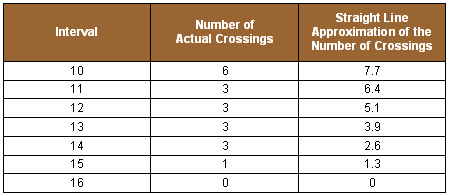Within each region above, we can readily determine what is the total number of possible crossing ELSs and the total number of possible ELSs using the formulas in the Table 4A. This is true because, in most cases: Within each region, each of these totals is of a sequence of numbers that either increases or decreases by the same amount from one interval to the next, or it stays constant. For the total number of possible crossings, each successive number is L more than the previous number in region R0 up until the interval equals the width (W). Then it remains constant for the rest of the region. The total number of crossings is the same for all intervals in region R1. For the total number of possible ELSs, each successive number is L less than the previous number throughout all regions. Column (C) of Table 3C provides an example of this phenomenon. We can quickly derive a formula for the total number of numbers of ELSs in each sequence. The sum of a series of numbers that increase or decrease by a constant amount is equal to the average of the first and last number of the sequence, times how many numbers there are in the sequence. As an example of the last formula, take the sequence of 1,3,5,7 and 9. The average of the first and last numbers is 5 (=(1 + 9)/2). Since there are 5 numbers, the total of this sequence is 5 * 5, or 25. This can be verified by adding up the numbers. This equality works because the average of the first and last numbers of the sequence is the same as the average of all the numbers � since they increase or decrease by a constant amount. And it is clearly true that the total of a sequence of numbers is equal to the average of all the numbers times how many numbers there are in the sequence. And, the average is nothing other than the total of all the numbers, divided by how many numbers there are. Once we have values for M0, M1 and M2, then the total number of numbers in each region can be obtained using the formulae: Table 4C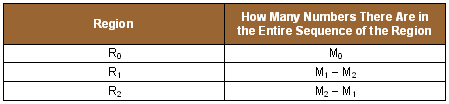We may recallSince the definition of region R0 is that i < (c / L), then the largest interval to be found in R0 is M0 = integer ( (c – 1) / L ). If the range of intervals had been defined with a "less than or equal to" rather than simply a "less than" � then our formula would have been M0 = integer ( c / L ). However, in the case where (c / L) is an exact whole number, then M0 must be the next smaller integer. This is accomplished using the above given formula. In a corresponding way, the formula for M1 is M1 = integer ( (d – 1) / L ). Finally, M2 is the same as the maximum possible interval (M) that was defined by Formula 1E. This results in the following formulas: Table 4D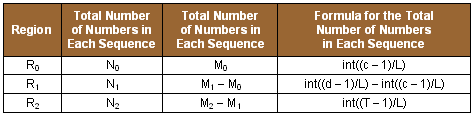Now we will go on to determine the average of the number of crossing ELSs within each region. Our starting point is a portion of an earlier table that gives us the formula for the number of possible crossing ELSs with a given interval (i). We have reproduced it below for ease of reference. Table 4E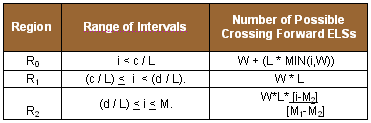We will now look at a table showing the first number in each region for the total number of crossing ELSs and the last number of crossings and the formula for the average of these two numbers. Table 4F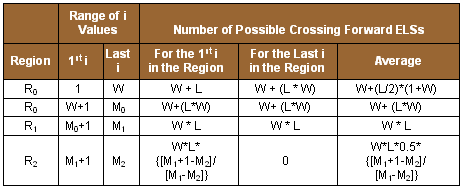If we multiply the third column of Table 4C by the last column of Table 4E for regions R1 and R2, we will get the following formulas for the number of possible crossing ELSs within each region: Table 4G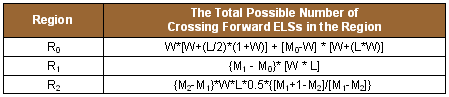The above formula for region R0 was also derived in the same way, except that it was necessary to split R0 into two ranges of i values. Again, while this may look fairly complex, these are actually fairly simple formulas to key into spreadsheet cells so that the probability of crossing can be quickly calculated. If we want to obtain the total number of possible crossing ELSs, including both forward and backward ELSs, then the formulas in Table 4F would need to be doubled. To finish our calculations, we only need to sum up all of the above numbers in Table 4F, calculate the total possible number of ELSs within the entire text using Formula 1C and take the ratio of these two numbers to get the desired probability. This probability is for the likelihood of a crossing ELS when the interval can be any number between 1 and the maximum possible interval within the entire text. We can also use the above table to quickly calculate similar probabilities when the maximum interval (N) is some number less than the maximum possible (M2). We first need to determine to which region N belongs. We will then substitute N for the maximum interval in that region (which could be either M0, M1or M2) and redo the formula above using N rather than that maximum interval. If N falls in either R0 or R1, then of course we will not include calculations from the subsequent region or regions. In the special case where N is less than W, then the total possible number of forward crossing ELSs is given by N*(W+(L/2)*(1+N)). There are N numbers in the sum of the number of crossing ELSs. The first is the number of crossing ELSs when the interval is 1 (W+L). The last is the number of crossing ELSs when the interval is N (W+L*N). Consequently, the sum of the number of crossing ELSs is the average of those numbers times the N numbers, or (N/2) * (W+L+W+L*N). This expression reduces down to N*(W+(L/2)*(1+N)). Again, this formula would need to be multiplied by 2 to determine the number of forward and backward crossing ELSs. This gives us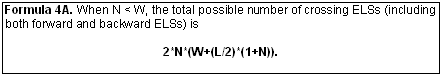Enjoy finding your own Bible codes. Bible code search software is available in our online store. Subscribe Free! By signing up to be a member of The Isaac Newton Bible Research Society, you will have access to more than fifteen years of research by our team of Bible code researchers. Sign up to be a member today.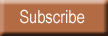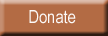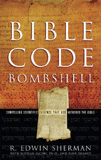Bombshell examines two massive, recently discovered clusters of codes in the Hebrew Old Testament. To read more about Bombshell, click here, or click below to order from Amazon today!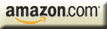| HOME | SUBSCRIBERS ONLY | OUR PURPOSE | FREE SAMPLES | CURRENT | | FAQs | ABOUT BIBLE CODES | SUBSCRIBE | CONTACT | Copyright © 2016 BibleCodeDigest.com